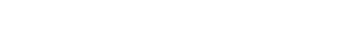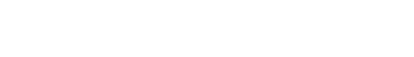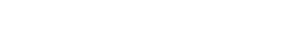#### 2. 数据不平衡处理方法

imbalanced-learn库提供了许多不平衡数据处理的方法，本文的例子都以imbalanced-learn库来实现。

`pip install -U imbalanced-learn`

https://github.com/scikit-learn-contrib/imbalanced-learn

```import pandas as pd
train_data = './data/train.csv'
test_data = './data/test.csv'
print(train_df.groupby(['label']).size())
# label为是否违约， 1为违约， 0为非违约
#     label
# 0    37243
# 1     2757```#### 2.1 欠采样```from imblearn.under_sampling import TomekLinks
X_train = train_df.drop(['id', 'type'], axis=1)
y = train_df['label']
X_us, y_us = tl.fit_sample(X_train, y)
print(X_us.groupby(['label']).size())
# label
# 0    36069
# 1     2757```

```from imblearn.under_sampling import ClusterCentroids
cc = ClusterCentroids(random_state=42)
X_res, y_res = cc.fit_resample(X_train, y)
X_res.groupby(['label']).size()
# label
# 0    2757
# 1    2757```

im-balance提供的欠采样的方法如下：

Random majority under-sampling with replacement
Under-sampling with Cluster Centroids
NearMiss-(1 & 2 & 3)
Condensed Nearest Neighbour
One-Sided Selection
Neighboorhood Cleaning Rule
Edited Nearest Neighbours
Instance Hardness Threshold
Repeated Edited Nearest Neighbours
AllKNN

#### 2.2 过采样SMOTE基于minority样本相似的特征空间构造新的人工样本。步骤如下：```from imblearn.over_sampling import SMOTE
smote = SMOTE(k_neighbors=5, random_state=42)
X_res, y_res = smote.fit_resample(X_train, y)
X_res.groupby(['label']).size()
# label
# 0    37243
# 1    37243``````from imblearn.over_sampling import BorderlineSMOTE
bsmote = BorderlineSMOTE(k_neighbors=5, random_state=42)
X_res, y_res = bsmote.fit_resample(X_train, y)
X_res.groupby(['label']).size()
# label
# 0    37243
# 1    37243``````from imblearn.over_sampling import ADASYN
X_res.groupby(['label']).size()
# label
# 0    37243
# 1    36690```

im-balance提供的过采样的方法如下（包括SMOTE算法的变种）：

Random minority over-sampling with replacement
SMOTE – Synthetic Minority Over-sampling Technique
SMOTENC – SMOTE for Nominal Continuous
bSMOTE(1 & 2) – Borderline SMOTE of types 1 and 2
SVM SMOTE – Support Vectors SMOTE
KMeans-SMOTE
ROSE – Random OverSampling Examples

#### 2.3 综合采样

```from imblearn.combine import SMOTETomek
smote_tomek = SMOTETomek(random_state=0)
X_res, y_res = smote_tomek.fit_sample(X_train, y)
X_res.groupby(['label']).size()
# label
# 0    36260
# 1    36260```

#### 2.4 模型集成

```from imblearn.ensemble import BalancedRandomForestClassifier
from sklearn.datasets import make_classification
X, y = make_classification(n_samples=1000, n_classes=3,
n_informative=4, weights=[0.2, 0.3, 0.5],
random_state=0)
clf = BalancedRandomForestClassifier(max_depth=2, random_state=0)
clf.fit(X, y)
print(clf.feature_importances_)
print(clf.predict([[0, 0, 0, 0, 0, 0, 0, 0, 0, 0,
0, 0, 0, 0, 0, 0, 0, 0, 0, 0]]))```

im-balance提供的模型集成的方法如下

Easy Ensemble classifier
Balanced Random Forest
Balanced Bagging
RUSBoost

#### 2.5 调整类别权重或者样本权重

```import lightgbm as lgb
clf = lgb.LGBMRegressor(num_leaves=31,
min_child_samples= np.random.randint(20,25),
max_depth=25,
learning_rate=0.1,
class_weight={0:1, 1:10},
n_estimators=500,
n_jobs=30)```